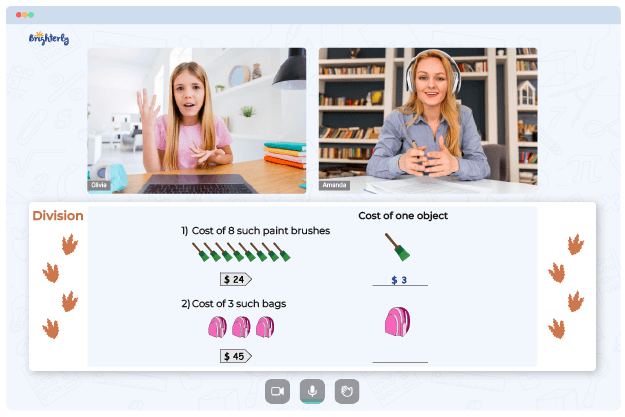# Multi Digit Division Worksheets

Mathematical worksheets have many uses as they can be utilized in assessment, drill, and instruction. During school vacations, parents can work with their kids on additional practice, teach them a new arithmetic skill, or keep a child’s abilities sharp. This paper covers the benefits of multi digit division worksheets and the reasons Brighterly’s tutors use them.

## Why Tutors from Brighterly use Multi Digit Division Worksheets

Brighterly is an online platform with professionals who help students understand math better. The tutors at Brughterly use math worksheets to help students become proficient in multi-digit division. They use a dividing multi digit numbers worksheet for practicing long division with divisors of varying digits, both with and without remainders.

Math for Kids

Is Your Child Struggling With Math?
1:1 Online Math TutoringThese dividing multi digit numbers worksheets assist students in getting comfortable with long division. The complexity of exercises progresses to more challenging questions as kids continue mastering multi-digit divisors.### Multi Digit Division Worksheets PDF

Dividing Multi Digit Numbers Worksheet### Multi Digit Division Worksheets PDF

Multi Digit Division Worksheet### Multi Digit Division Worksheets PDF

Dividing Multi Digit Numbers Worksheets### Multi Digit Division Worksheets PDF

Divide Multi Digit Numbers Worksheet

The divide multi digit numbers worksheet introduces learners to division fact drills and lengthy division practice, both with and without remainders. Using a multi digit division worksheet, kids can enjoy the learning process without the monotonousness of conventional math practices.

### More Division Worksheets

Need help with Division?• Does your child need extra assistance with mastering the concept of division?
• Start learning with an online tutor.

Is your child finding it hard to understand of division? An online tutor could be of help.